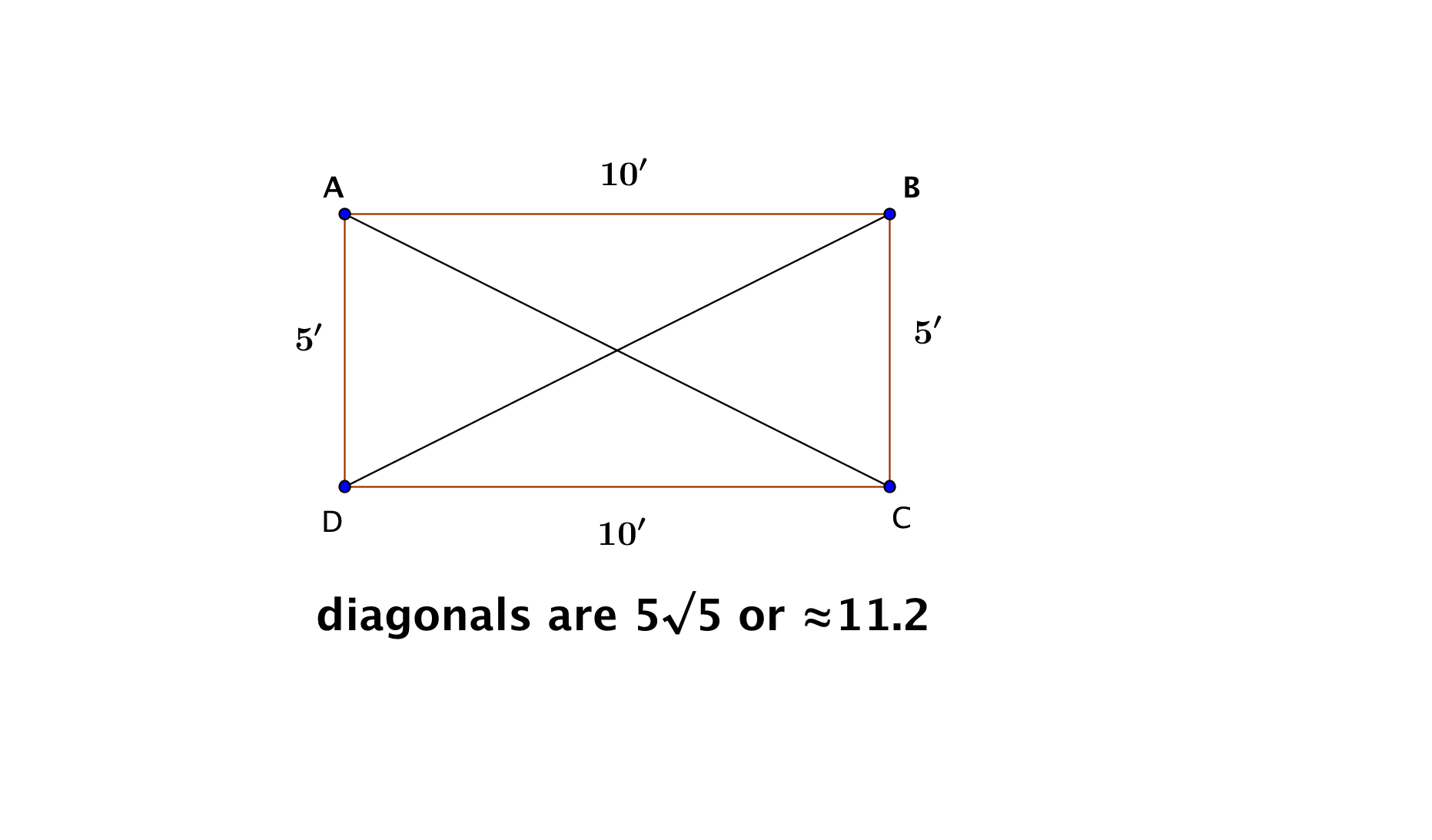# GEOM 3 | Lesson 2 | Try This! Solution

1. Note that if the diagonals are the same length, then $$ΔADC ≅ ΔBCD$$ similarly $$ΔADC ≅ ΔABC$$, that means that the angles: $$∠DAB,∠ABC, ∠BCD, ∠ADC$$ are all the same size or 90˚.1. The popsicle sticks would need to be all the same size to form the equilateral triangles.
2. The measurements of the parts of the isosceles triangles to ensure that the two sides sides are congruent, also the measurement of the base is needed.  Using the sides of one of the right triangles, check using the pythagorean theorem to ensure that the sum of the squares of the legs is equal to the square of the hypotenuse.  If it is then you have a right triangle.
3.The top and the bottom piece need to be $$100’$$.  There are $$8$$ rectangles, which means that they must be $$12.5’$$ wide and they are $$10$$.  The cross beam needs to be $$\sqrt{12.5^2+10^2}$$ or $$16’$$.